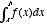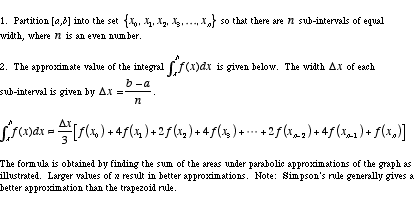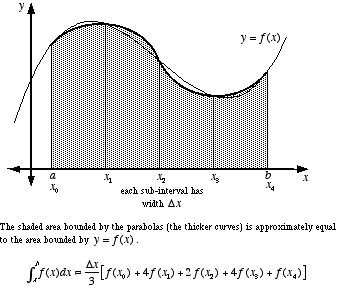index: click on a letter A B C D E F G H I J K L M N O P Q R S T U V W X Y Z A to Z index index: subject areas numbers & symbols sets, logic, proofs geometry algebra trigonometry advanced algebra & pre-calculus calculus advanced topics probability & statistics real world applications multimedia entrieswww.mathwords.com about mathwords website feedback

 Simpson's Rule A method for approximating a definite integralusing parabolic approximations of f. The parabolas are drawn as shown below. To use Simpson's rule follow these two steps:See also